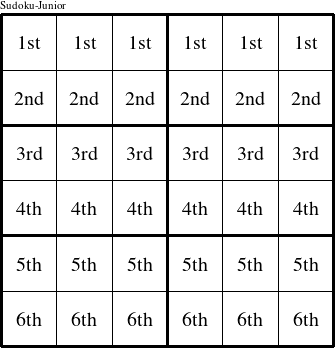#### Printable version

Detailed instructions for working a group puzzle of type 'Sudoku-Junior'

The 6 elements in each group of this type of puzzle are: 1, 2, 3, 4, 5, and 6.

Every group in the puzzle must contain exactly this set of elements.

####In a Sudoku-Junior puzzle, each row is a group numbered as shown in the figure above.

####In a Sudoku-Junior puzzle, each column is a group numbered as shown in the figure above.

####In a Sudoku-Junior puzzle, each 3x2 rectangle is a group numbered as shown in the figure above.